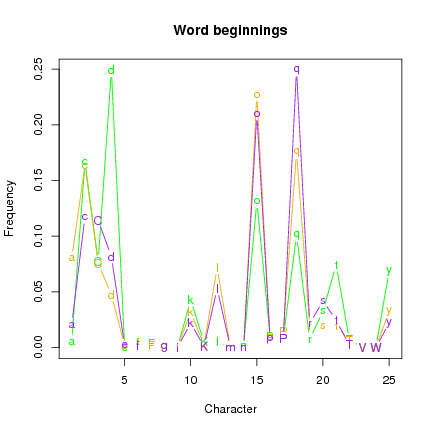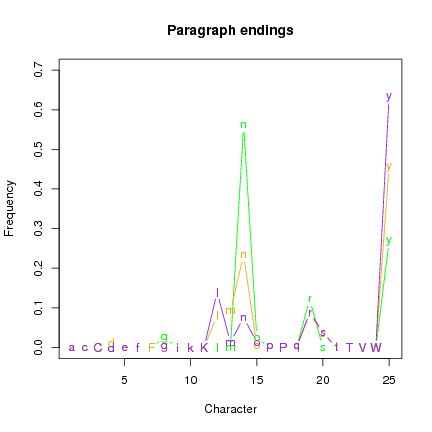# Voynich Manuscript - Patterns of Co-occurrence

## 1  Introduction

These analyses continue using the three discrete subsets described in the first page: an herbal subset (H) in Currier language A, and the recipe section (R) and the balenological section (B), both in Currier language B.
Having found differences in character frequencies, I also wanted to look at patterns of word beginnings and endings, and paragraph beginnings and endings. (Note that "capital gallows characters" are by definition paragraph-initial.)Figure 1: Frequencies of characters beginning words.
Set H is the only group where d was an extremely frequent word-initial character, and t and y were also more frequent in this position in set H than the other two (Fig. 1). The characters o, q and especially l were less common as word-initials in set H. Sets R and B were similar, although there are differences in the frequency of a and d between the two sets.Figure 2: Frequencies of characters beginning paragraphs.
Paragraph-initial characters were mainly the gallows characters F, K, P, T, and q and o were also found (Fig. 2). In set R, d was found as a paragraph-initial character. F and K were more common in set H, and P was less common.Figure 3: Frequencies of characters ending words.
Word-ending characters were similar in all three sets, and only four characters were commonly found: l, n, r and y (Fig. 3). The same characters were found in the paragraph-final position, but frequencies among sets were more variable (Fig. 4).Figure 4: Frequencies of characters ending paragraphs.